Search IntMath
Close

# Microsoft Mathematics 4.0 - now for $0 By Murray Bourne, 17 Aug 2011 I reviewed Microsoft Math 3.0 a while back. It was quite good, even though it had some bugs (and it cost just under$20).

The new release, Microsoft Mathematics 4.0, comes for free. There are 2 flavors of MS Mathematics 4.0:

1. The standalone version (requires either Windows XP, Vista, Windows 7 or later and .NET framework, which is also a free download).
2. The add-in version, which only works with Microsoft Word 2010, Microsoft OneNote 2010, and Microsoft Office Word 2007. The add-in allows you to easily insert math objects (graphs, equations, etc) into Word or OneNote.

The Add-In is invoked from the ribbon in MS Word. It's reasonably well integrated, but there are a few usability gripes, as mentioned below.

The current version seems to do everything that Microsoft Math 3.0 did before. As the site says:

• Compute standard mathematical functions, such as roots and logarithms
• Compute trigonometric functions, such as sine and cosine
• Find derivatives and integrals, limits, and sums and products of series
• Perform matrix operations, such as inverses, addition, and multiplication
• Perform operations on complex numbers
• Plot 2-D graphs in Cartesian and polar coordinates
• Plot 3-D graphs in Cartesian, cylindrical, and spherical coordinates
• Solve equations and inequalities
• Calculate statistical functions, such as mode and variance, on lists of numbers
• Factor polynomials or integers
• Simplify or expand algebraic expressions

Like all such software, you need to know what you can ask it to do, and how to ask it. It won't directly solve your word problems - you still need to use your brains for that!

## So is MS Math 4.0 any good?

MS Math does a good job of graphing 2-D and 3-D graphs.

For example, here is a paraboloid. You can easily draw a plane intersecting the 3-D surface (the plane is light blue on the left of the diagram below and the intersection of the plane is a parabola, in dark blue). This is quite a useful feature, but only exists in the Add-In version.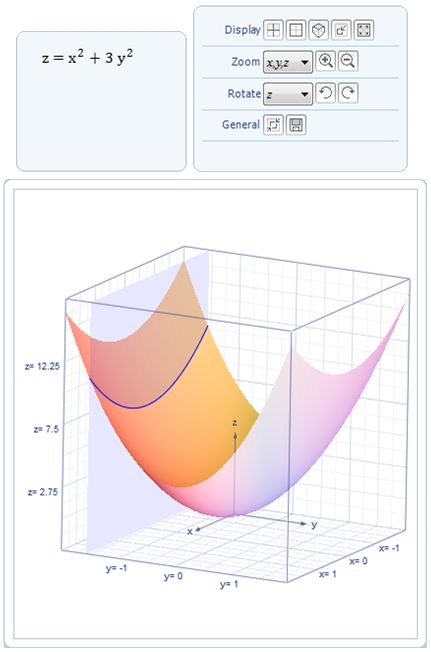A paraboloid graphed using MS Math 4

## How does MS Math 4 graph arccot(x)?

I wrote recently about the fact that different math software disagree on the correct graph for y = arccot(x). (That is, the inverse of the reciprocal of tan(x)). Here's the article:

Which is the correct graph of arccot x?

Microsoft Mathematics 4.0 interprets the graph in the following way, the same as Mathematica and Matlab, but not the same as Mathcad's version.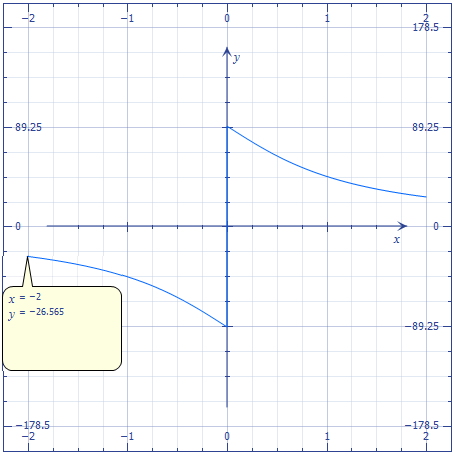MS Math 4's graph of y = arccot(x), showing the Trace facility

In the above graph, you can see the "trace" facility in use. By clicking on the Trace forward arrow on the graph in MS Math, you can move along the graph as x takes values from smaller to larger numbers.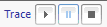## Solving Equations

You can easily solve equations using Microsoft Math 4.0, as you could earlier in MS MAth 3.0, as the following example shows: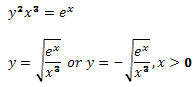Micrsoft's choice of font in MS Math 4 results in quite unreadable text at times. Believe it or not, that's xraised to the power 3 in the above example.

## Inverse of a Matrix

Inverses of matrices are possible, too.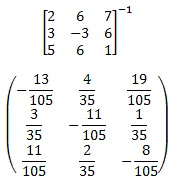## One gripe

Next I tried out numerical integration, this time using the Word Add-In. This is what I asked Mathematics 4.0 to calculate: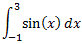What I got was the following error message: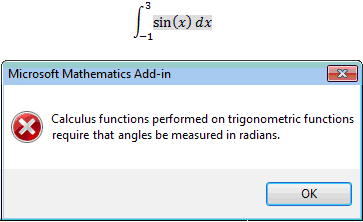No argument with the requirement to change to radians, but I then needed to go back a few steps, and change the setting to radians, as follows: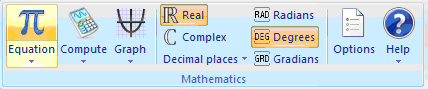Then I needed to enter the question again, and finally it gave me the answer: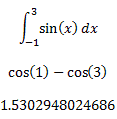How about some usability testing, Microsoft? It doesn't take much to change the warning dialog box to include an option "Would you lilke to change to radians now?".

Or even better, it could just change to radians automatically, and alert the reader what happened. (This is what happens in the standalone version, as it should.)

## Should you get both the standalone and the Add-In for Word and OneNote versions?

Personally, I'd stick to the stand-alone MS Mathematics 4.0 application, since you don't need Word or OneNote to use it. You can always copy-paste into Word or OneNote.

However, the image you get after pasting from the standalone version is really poor quality and there seems to be more features in the Add-In version.

## Conclusion

This is a very good product, especially for the price ("free" after purchasing Windows and Word). Microsoft has finally figured out that it's good business to give away stuff.

It's certainly worth adding MS Math 4 to your kit of math tools.

### Comment Preview

HTML: You can use simple tags like <b>, <a href="...">, etc.

To enter math, you can can either:

1. Use simple calculator-like input in the following format (surround your math in backticks, or qq on tablet or phone):
a^2 = sqrt(b^2 + c^2)
(See more on ASCIIMath syntax); or
2. Use simple LaTeX in the following format. Surround your math with $$ and $$.
$$\int g dx = \sqrt{\frac{a}{b}}$$
(This is standard simple LaTeX.)

NOTE: You can mix both types of math entry in your comment.